Documentation

## Antenna Modeling and Analysis

This example shows how to construct, visualize and analyze the antenna elements in the Antenna Toolbox.

### Define Antenna Element Using the Antenna Library

Define a helix antenna using the `helix` antenna element in the Antenna Modeling and Analysis library.

```hx = helix ```
```hx = helix with properties: Radius: 0.0220 Width: 1.0000e-03 Turns: 3 Spacing: 0.0350 WindingDirection: 'CCW' FeedStubHeight: 1.0000e-03 GroundPlaneRadius: 0.0750 Tilt: 0 TiltAxis: [1 0 0] Load: [1x1 lumpedElement] ```

### Show Structure of Antenna

Use the `show` function to view the structure of the helix antenna. A helical antenna consists of a helical shaped conductor on a ground plane. The ground plane of the antenna is in the X-Y plane.

```show(hx) ```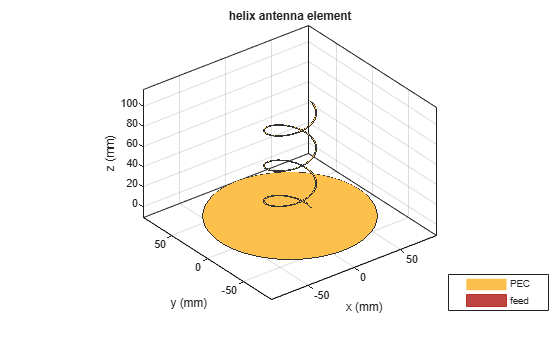### Modify Properties of Antenna

Modify the following properties of the helix antenna: Radius = 28e-3, Width = 1.2e-3, Number of Turns = 4 Display the properties of the antenna. View the antenna to see the change in structure.

```hx = helix('Radius',28e-3,'Width',1.2e-3,'Turns',4) show(hx) ```
```hx = helix with properties: Radius: 0.0280 Width: 0.0012 Turns: 4 Spacing: 0.0350 WindingDirection: 'CCW' FeedStubHeight: 1.0000e-03 GroundPlaneRadius: 0.0750 Tilt: 0 TiltAxis: [1 0 0] Load: [1x1 lumpedElement] ```### Plot Radiation Pattern of Antenna

Use `pattern` function to plot the radiation pattern of the helix antenna. The radiation pattern of an antenna is the spatial distribution of power of an antenna. The pattern displays the directivity or gain of the antenna. By default, the pattern function plots the directivity of the antenna.

```pattern(hx,1.8e9) ```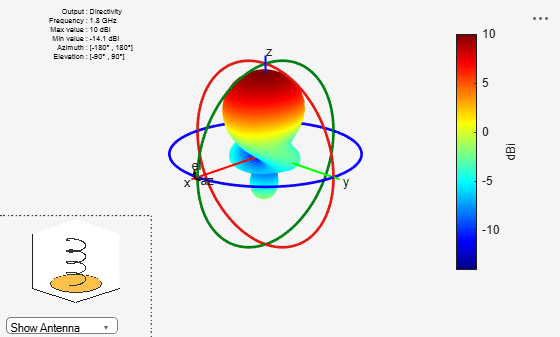### Plot Azimuth and Elevation Pattern of Antenna

Use `patternAzimuth` and `patternElevation` functions to plot the azimuth and elevation pattern of the helix antenna. This is the 2D radiation pattern of the antenna at a specified frequency.

```patternAzimuth(hx,1.8e9) figure patternElevation(hx,1.8e9) ```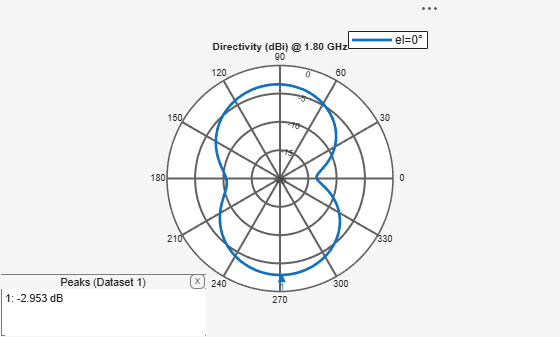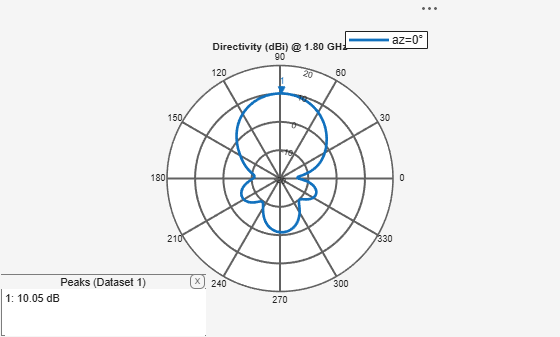### Calculate Directivity of Antenna

Use Directivity name-value pair in the output of the pattern function to calculate the directivity of helix antenna. Directivity is the ability of an antenna to radiate power in a particular direction. It can be defined as ratio of maximum radiation intensity in the desired direction to the average radiation intensity in all other directions.

```Directivity = pattern(hx,1.8e9,0,90) ```
```Directivity = 10.0430 ```

### Calculate EHfields of Antenna

Use the `EHfields` function to calculate the EH fields of the helix antenna. EH fields are the x, y, z components of electric and magnetic fields of an antenna. These components are measured at a specific frequency and at specified points in space.

```[E,H] = EHfields(hx,1.8e9,[0;0;1]) ```
```E = -0.5241 - 0.5727i -0.8760 + 0.5252i -0.0036 + 0.0006i H = 0.0023 - 0.0014i -0.0014 - 0.0015i 0.0000 - 0.0000i ```

### Plot Different Polarizations of Antenna

Use the Polarization name-value pair in the pattern function to plot the different polarization patterns of the helix antenna. Polarization is the orientation of the electric field, or E-field, of an antenna. Polarization is classified as elliptical, linear, or circular. This example shows the Right-Hand Circularly Polarized(RHCP) radiation pattern of the helix.

```pattern(hx,1.8e9,'Polarization','RHCP') ```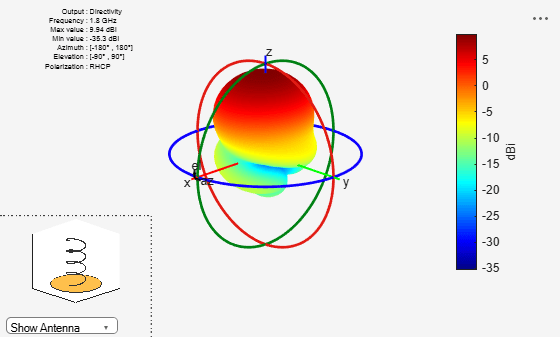### Calculate Axial Ratio of Antenna

Use the `axialRatio` function to calculate the axial ratio of the helix antenna. Antenna axial ratio (AR) in a given direction quantifies the ratio of two orthogonal field components radiated in a circularly polarized wave. An axial ratio of infinity, implies a linearly polarized wave. The unit of measure is dB.

```ar = axialRatio(hx,1.8e9,20,30) ```
```ar = 23.6238 ```

### Calculate Beamwidth of Antenna

Use the `beamwidth` function to calculate the beamwidth of the antenna. Antenna beamwidth is the angular measure of the antenna pattern coverage. Beamwidth angle is measured in plane containing the direction of main lobe of the antenna.

```[bw, angles] = beamwidth(hx,1.8e9,0,1:1:360) ```
```bw = 57.0000 angles = 60 117 ```

### Calculate Impedance of Antenna

Use the `impedance` function to calculate and plot the input impedance of helix antenna. Input impedance is a ratio of voltage and current at the port. Antenna impedance is calculated as the ratio of the phasor voltage (which is 1V at a phase angle of 0 deg as mentioned earlier) and the phasor current at the port.

```impedance(hx,1.7e9:1e6:2.2e9) ```### Calculate Reflection Coefficient of Antenna

Use the `sparameters` function to calculate the S11 of the helix antenna. Antenna reflection coefficient, or S_1_1, describes a relative fraction of the incident RF power that is reflected back due to the impedance mismatch.

```S = sparameters(hx,1.7e9:1e6:2.2e9,72) rfplot(S) ```
```S = sparameters: S-parameters object NumPorts: 1 Frequencies: [501x1 double] Parameters: [1x1x501 double] Impedance: 72 rfparam(obj,i,j) returns S-parameter Sij ```### Calculate Return Loss of Antenna

Use the `returnLoss` function to calculate and plot the return loss of the helix antenna. Antenna return loss is a measure of the effectiveness of power delivery from a transmission line to a load such as antenna. The calculations are displayed in logscale.

```returnLoss(hx,1.7e9:1e6:2.2e9,72) ```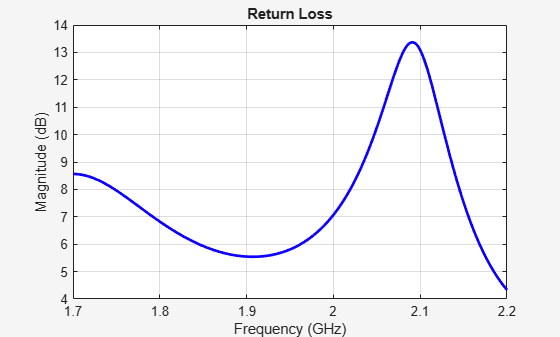### Calculate Voltage Standing Wave Ratio (VSWR) of Antenna

Use the `vswr` function to calculate and plot the VSWR of the helix antenna. The antenna VSWR is another measure of impedance matching between transmission line and antenna.

```vswr(hx,1.7e9:1e6:2.2e9,72) ```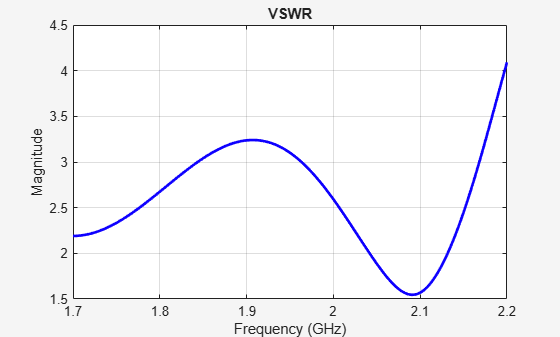### Calculate Current and Charge Distribution of Antenna

Use the `charge` function to calculate the charge distribution of the helix antenna. Charge distribution is the value of charge on the antenna surface at a specified frequency. Use the `current` function to calculate the current distribution of the helix antenna. Current distribution is the value of current on the antenna surface at a specified frequency.

```charge(hx,2.01e9) figure current(hx,2.01e9) ```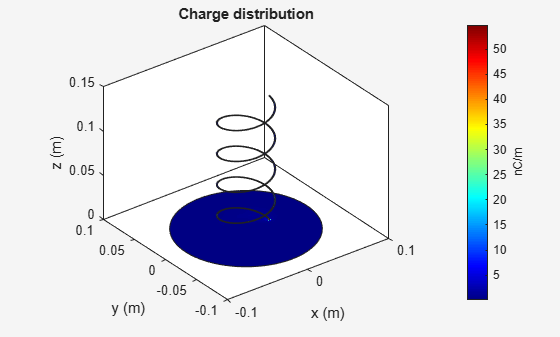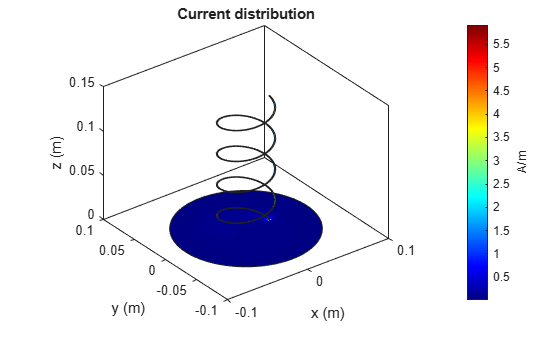### Show Mesh of Antenna

Use the `mesh` function to create and show a mesh structure of the helix antenna. mesh is used to discretize antenna surface. In this process, the electromagnetic solver can process the geometry and material of the antenna. The shape of the basis or the discretizing element for subdividing the antenna surface is a triangle.

```mesh(hx) ```### Mesh Antenna Manually

Specify the maximum edge length for the triangles using the 'MaxEdgeLength' name-value pair. This name-value pair meshes the helix structure manually.

```mesh(hx,'MaxEdgeLength',0.01) ```### Change Meshing to Automatic

```meshconfig(hx,'auto') ```
```ans = struct with fields: NumTriangles: 890 NumTetrahedra: 0 NumBasis: [] MaxEdgeLength: 0.0100 MeshMode: 'auto' ```

Please find the link to Antenna Near Field visualization example below: Antenna Near-Field Visualization

### References

 Balanis, C.A. "Antenna Theory. Analysis and Design", p. 514, Wiley, New York, 3rd Edition, 2005.